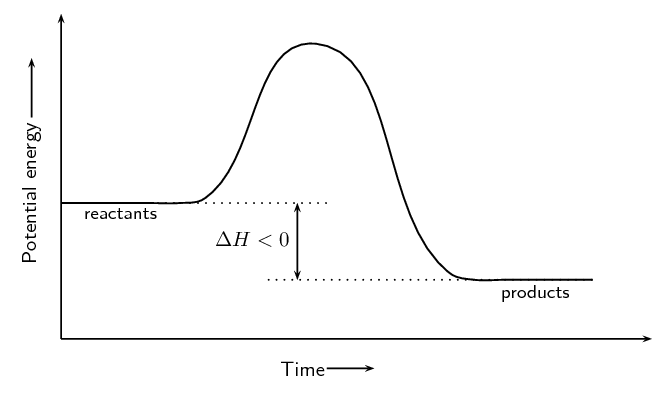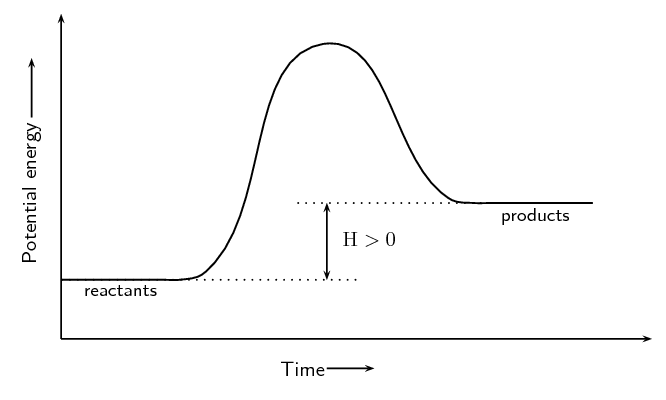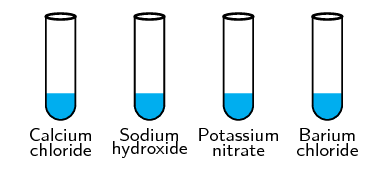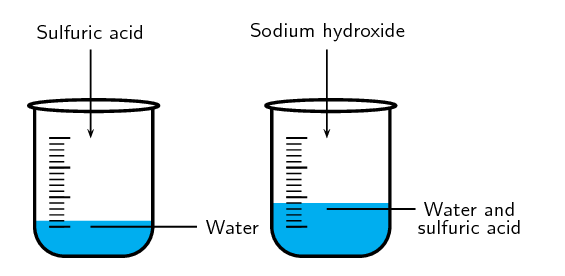Home Practice
For learners and parents For teachers and schools
Textbooks
Full catalogue
Pricing SupportLog in

We think you are located in United States. Is this correct?

# 12.2 Exothermic and endothermic reactions

## 12.2 Exothermic and endothermic reactions (ESBQP)

### The heat of reaction (ESBQQ)

The heat of the reaction is represented by the symbol $$Δ\text{H}$$, where:

$Δ \text{H} = E_{\text{prod}} - E_{\text{react}}$

• In an exothermic reaction, $$Δ \text{H}$$ is less than zero because the energy of the reactants is greater than the energy of the products. Energy is released in the reaction. For example:

$\text{H}_{2}\text{(g)} + \text{Cl}_{2}\text{(g)} → 2\text{HCl(g)} \qquad Δ\text{H} < 0$
• In an endothermic reaction, $$Δ \text{H}$$ is greater than zero because the energy of the reactants is less than the energy of the products. Energy is absorbed in the reaction. For example:

$\text{C (s)} + \text{H}_{2}\text{O(ℓ)} → \text{CO(g)} + \text{H}_{2}\text{(g)} \qquad Δ\text{H} > 0$

Some of the information relating to exothermic and endothermic reactions is summarised in Table 12.1.

 Type of reaction Exothermic Endothermic Energy absorbed or released Released Absorbed Relative energy of reactants and products Energy of reactants greater than energy of product Energy of reactants less than energy of product Sign of ΔH Negative (i.e. $$< 0$$) Positive (i.e. $$> 0$$)
Table 12.1: A comparison of exothermic and endothermic reactions.

Writing equations using ΔH

ΔH has been calculated for many different reactions and so instead of saying that ΔH is positive or negative, we can look up the value of ΔH for the reaction and use that value instead.

There are two ways to write the heat of the reaction in an equation. For the exothermic reaction $$\text{C(s)} + \text{O}_{2}\text{(g)} → \text{CO}_{2}\text{(g)}$$, we can write:

$\text{C(s)} + \text{O}_{2}\text{(g)} → \text{CO}_{2}\text{(g)} \qquad Δ\text{H} = -\text{393}\text{ kJ·mol^{-1}}$

or

$\text{C(s)} + \text{O}_{2}\text{(g)} → \text{CO}_{2}\text{(g)} + \text{393}\text{ kJ·mol^{-1}}$

For the endothermic reaction, $$\text{C(s)} + \text{H}_{2}\text{O(g)} → \text{H}_{2}\text{(g)} + \text{CO(g)}$$, we can write:

$\text{C(s)} + \text{H}_{2}\text{O(g)} → \text{H}_{2}\text{(g)} + \text{CO(g)} \qquad Δ\text{H} = \text{+131}\text{ kJ·mol^{-1}}$

or

$\text{C(s)} + \text{H}_{2}\text{O(g)} + \text{+131}\text{ kJ·mol^{-1}} → \text{H}_{2}\text{(g)} + \text{CO(g)}$

The units for ΔH are $$\text{kJ·mol^{-1}}$$. In other words, the ΔH value gives the amount of energy that is absorbed or released per mole of product that is formed. Units can also be written as $$\text{kJ}$$, which then gives the total amount of energy that is released or absorbed when the product forms.

The energy changes during exothermic and endothermic reactions can be plotted on a graph:Figure 12.2: The energy changes that take place during an exothermic reaction.Figure 12.3: The energy changes that take place during an endothermic reaction.

We will explain shortly why we draw these graphs with a curve rather than simply drawing a straight line from the reactants energy to the products energy.

In the investigation on exothermic and endothermic reactions learners work with concentrated sulfuric acid. They must work in a well ventilated room or, if possible, in a fume cupboard. This is a highly corrosive substance and learners must handle it with care. If they spill any on themselves they must immediately wash the affected area with plenty of running water. Either the learner or their friend should inform you as soon as possible so you can ensure that the learner is ok. If necessary the learner may need to go to the bathroom to remove and rinse clothing that is affected. Either you or another learner should accompany them.

Most of the salts that the learners will work with are hygroscopic and will quickly absorb water from the air. These salts can cause chemical burns and should be handled with care. If possible learners should wear gloves to protect their hands.

## Endothermic and exothermic reactions

### Aim

To investigate exothermic and endothermic reactions.

### Apparatus and materials

• Approximately $$\text{2}$$ $$\text{g}$$ of calcium chloride $$(\text{CaCl}_{2})$$
• Approximately $$\text{2}$$ $$\text{g}$$ of sodium hydroxide $$(\text{NaOH})$$
• Approximately $$\text{2}$$ $$\text{g}$$ of potassium nitrate $$(\text{KNO}_{3})$$
• Approximately $$\text{2}$$ $$\text{g}$$ of barium chloride $$(\text{BaCl}_{2})$$
• concentrated sulphuric acid $$(\text{H}_{2}\text{SO}_{4})$$ (Be careful, this can cause serious burns)
• 5 test tubes
• thermometer

When working with concentrated sulfuric acid always wear gloves and safety glasses. Always work in a well ventilated room or in a fume cupboard.

### Method1. Dissolve about $$\text{1}$$ $$\text{g}$$ of each of the following substances in $$\text{5}$$-$$\text{10}$$ $$\text{cm^{3}}$$ of water in a test tube: $$\text{CaCl}_{2}$$, $$\text{NaOH}$$, $$\text{KNO}_{3}$$ and $$\text{BaCl}_{2}$$.

2. Observe whether the reaction is endothermic or exothermic, either by feeling whether the side of the test tube gets hot or cold, or using a thermometer.

3. Dilute $$\text{3}$$ $$\text{cm^{3}}$$ of concentrated $$\text{H}_{2}\text{SO}_{4}$$ in $$\text{10}$$ $$\text{cm^{3}}$$ of water in the fifth test tube and observe whether the temperature changes.

Remember to always add the acid to the water.

4. Wait a few minutes and then carefully add $$\text{NaOH}$$ to the diluted $$\text{H}_{2}\text{SO}_{4}$$. Observe any temperature (energy) changes.

### Results

Record which of the above reactions are endothermic and which are exothermic.

 Exothermic reactions Endothermic reactions
• When $$\text{BaCl}_{2}$$ and $$\text{KNO}_{3}$$ dissolve in water, they take in heat from the surroundings. The dissolution of these salts is endothermic.

• When $$\text{CaCl}_{2}$$ and $$\text{NaOH}$$ dissolve in water, heat is released. The process is exothermic.

• The reaction of $$\text{H}_{2}\text{SO}_{4}$$ and $$\text{NaOH}$$ is also exothermic.

## Endothermic and exothermic reactions

Textbook Exercise 12.2

In each of the following reactions, say whether the reaction is endothermic or exothermic, and give a reason for your answer. Draw the resulting energy graph for each reaction.

$$\text{H}_{2}\text{(g)} + \text{I}_{2}\text{(g)} → 2\text{HI (g)} + \text{21}\text{ kJ·mol^{-1}}$$

Exothermic. Heat is given off and this is represented by showing $$+$$ energy on the right hand side of the equation.$$\text{CH}_{4}\text{(g)} + 2\text{O}_{2}\text{(g)} → \text{CO}_{2}\text{(g)} + 2\text{H}_{2}\text{O (g)} \qquad Δ\text{H} = -\text{802}\text{ kJ·mol^{-1}}$$

Exothermic. $$\Delta H$$ is negative for this reaction.The following reaction takes place in a flask:

$$\text{Ba(OH)}_{2}\cdot 8\text{H}_{2}\text{O(s)} + 2\text{NH}_{4}\text{NO}_{3}\text{(aq)} → \text{Ba(NO}_{3}\text{)}_{2}\text{(aq)} + 2\text{NH}_{3}\text{(aq)} + 10\text{H}_{2}\text{O(ℓ)}$$

Within a few minutes, the temperature of the flask drops by approximately $$\text{20}$$$$\text{°C}$$.

Endothermic. The temperature of the reaction vessel decreases indicating that heat was needed for the reaction.$$2\text{Na(aq)} + \text{Cl}_{2}\text{(aq)} → 2\text{NaCl(aq)} \qquad Δ\text{H} = -\text{411}\text{ kJ·mol^{-1}}$$

Exothermic. $$\Delta H < 0$$$$\text{C(s)} + \text{O}_{2}\text{(g)} → \text{CO}_{2}\text{(g)}$$

Exothermic. This reaction is the combustion of wood or coal to form carbon dioxide and gives off heat.For each of the following descriptions, say whether the process is endothermic or exothermic and give a reason for your answer.

evaporation

Endothermic. Energy is needed to break the intermolecular forces.

the combustion reaction in a car engine

Exothermic. Energy is released.

bomb explosions

Exothermic. In an explosion a large amount of energy is released.

melting ice

Endothermic. Energy is needed to break the intermolecular forces.

digestion of food

Exothermic. Digestion of food involves the release of energy that your body can then use.

condensation

Exothermic. Energy is given off as the particles are going from a higher energy state to a lower energy state.

When you add water to acid the resulting solution splashes up. The beaker also gets very hot. Explain why.

The reaction between acid and water is an exothermic reaction. This reaction produces a lot of heat and energy which causes the resulting solution to splash. Since the acid is usually more dense than the water adding water to the acid causes the reaction to happen in a small area and on the surface. This leads to a more vigorous (fast) reaction. If you add the acid to the water then there is a larger volume of water to absorb the heat of the reaction and so the reaction proceeds more slowly.